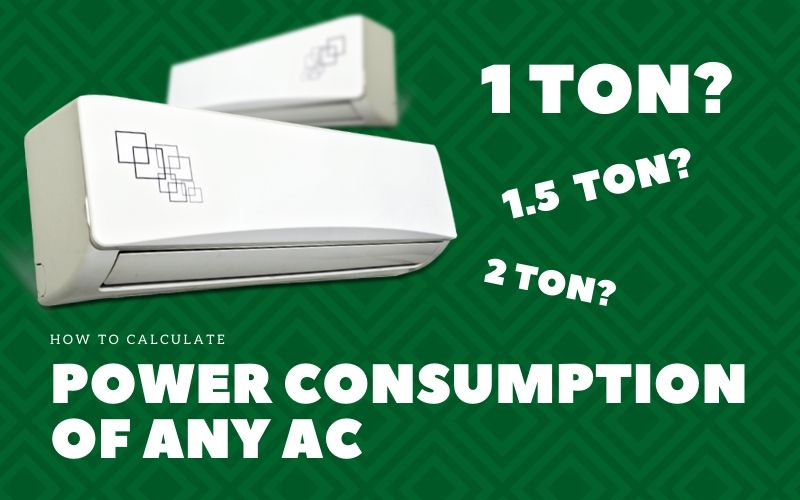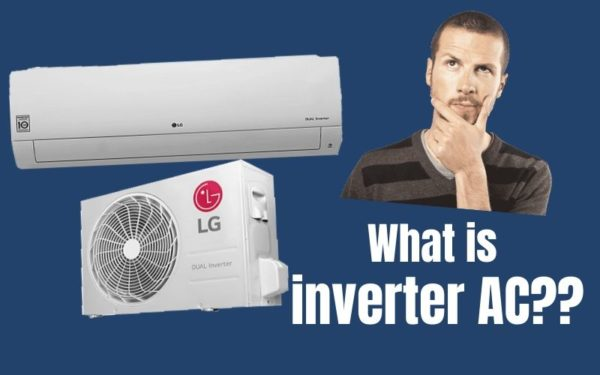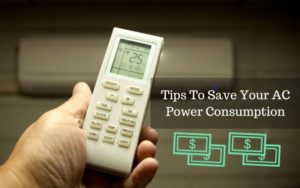## Air conditioner Power Consumption Calculator:Air conditioners are broadly of two types Inverter AC & non-inverter AC and power consumption of both these types of AC is different. In a conventional non-inverter AC the compressor is a single speed compressor which runs at maximum speed to cool the room and once the room is cooled it turns OFF and starts again when the room temperature goes above the set temperature ultimately the compressor of non-inverter AC runs at maximum speed irrespective of the cooling demand.

Whereas an inverter AC has variable speed compressor which adjusts its speed according to the load on your AC and cooling demands. In inverter AC compressor is always ON. An inverter AC is more energy efficient than conventional AC hence inverter air conditioner power consumption less than conventional air conditioner of same size.

To know more about inverter AC check out this blog What is inverter AC?

What is Watt (W), Kilowatt (KW), Kilowatt hour (kWh) (unit) of electricity?To use the air conditioner power consumption calculator to calculate the power consumption of your AC and interpret the results correctly you should understand the following terms.

If you know these terms then feel free to scroll down to use the AC power consumption calculator.

• What is Watt (W) – Watt is a unit of power. It means the rate at which electricity is consumed or produced by a device. For example a 250 watt refrigerator consumes power at a rate of 150 watts per hour, it does not mean that the refrigerator consumed 250 units of electricity, it means it will consume power at a rate of 250 watts every hour.
• What is Kilowatt (kW) – Kilowatt is also a unit of power. Kilowatt (KW) is used for large devices that consume more power. For example a 1.5 kW air conditioner, 6 kW elevator. There are bigger units like Megawatt (MW), Gigawatt (GW) to describe large power consumption or generation. For example 100 Megawatt (MW) coal power plant.(1 kW = 1000 W)
• What is a Kilowatt hour (kWh) – Units as described in electricity bill a.k.a kilowatt hour is the energy consumption of a device. For example a 1000 watt AC running for 5 hours will consume 1000 watt x 5 hours = 5000 watt hours = 5 kilowatt hours of electricity = 5 units of electricity.(1 kWh of electricity = 1 unit of electricity)
• Electricity Tariff – Electricity tariff is the amount the electricity provider charges you for one unit (kWh) of electricity. I live in Mumbai, India here the electricity tariff is Rs 12/kWh. If you don’t know how to calculate your tariff then just divide your total bill by monthly power consumption and you will get your electricity tariff.
• What is operational hours – Operational hours of a device refers to how many hours the device is working. If you run your television for 3 hours then operational hours of your television is 3 hours.

To calculate the power consumption of your AC just input the rated power of your AC, operation hours of your AC and the electricity tariff in your place.

A a rule of thumb, to calculate energy consumption consider 1 ton of AC is equal to 1000 watts.

So if you have 1.5 ton AC type 1500 watts in rated power section.

### How to interpret results:

Mind you the values obtained from the calculator will be almost twice the actual values since the compressor of non-inverter AC turns on and off depending on the cooling needs. On an average an AC compressor duty cycle is 50 % so divide your final answer by 2 and that should be your AC power consumption and corresponding electricity bill.

If you have an inverter AC still divide the answer by 2 as half of the time compressor is running at the slowest speed.

Note: The calculated result is approximate value to know your AC exact power consumption read this blog on Power Consumption of AC.

If your AC power consumption is high then follow this tips to reduce your AC power consumption and electricity bill.

## 10 Best ways to Reduce AC Power Consumption:

1. Set thermostat at 24 degree Celsius.
2. Clean AC filters periodically.
3. Keep the air conditioner room sealed.
4. Turn off appliances in your air conditioned room if not in use.
6. Make sure your indoor AC unit doesn’t get direct sunlight.
7. Use ceiling fans for proper air circulation.
8. Keep closet & other doors closed.

### Check out related blogs:## What is inverter AC?## How To Save Electricity in AC?

If you are feeling the pinch of high electricity bill...